The masterpieces of Leo Fender were created without the assistance of SPICE simulation or software analysis tools. Even a simple calculator with multiply and divide didn't become widespread until the 1970s. In 1973, the cost of upgrading to a model with a square root button would set you back more than a Pickering cartridge for your top-of-the-line Garrard Zero 100SB turntable!

The 1950s and 1960s were pinnacle of pencil, paper, and slide rule. Leo Fender, Jim Marshall, and Dave Reeves developed their designs without digital computers and transistor-bloated calculators. If they used a "computer" it would have been pure low-tech analog. With this tutorial I'm hoping that perhaps someone many years younger than I will pick up their grandfather's well-worn slide rule and experience an important aspect of the engineering environment in the era of the 5F6-A, the Plexi, and the DR103.

#### How to Read the Scales on a Slide Rule

Reading the scales of a slide rule is a lot like reading a ruler, except that the distances between marks are not uniform. Consider, for example, the C scale.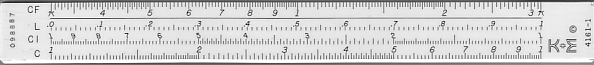There are 10 primary marks: 1, 2, 3, 4, 5, 6, 7, 8, 9, and 1. Between 2 and 3 there are secondary marks at 21, 22, 23, ..., 28, 29, and additional marks at 205, 215, 225, etc.New! Guitar Amplifier Electronics: Fender Deluxe - from TV front to narrow panel to brownface to blackface Reverb

Notice that I haven't used any decimal points. You would think that halfway between 2 and 3 would be 2.5, just like on a ruler. Instead I refer to this point as 25 without a decimal point. This is a clue to an important concept: the decimal point is left to you. The slide rule gives you the most significant digits but tells you nothing about the placement of the decimal point. Consider, for example,

(2.19)(23.1) = ?

You can't compute the exact value in your head, but you can guess that the answer is slightly more than 46. You know it's much smaller than 460 and it's much greater than 4.6. The human brain is accurate enough to keep track of the decimal point. It's the finer details that elude us. When the slide rule indicates the answer is the mark 215, it could represent 2.15 megohms, 215 volts, or 215 milliamps depending on the decimal point that we track in our heads.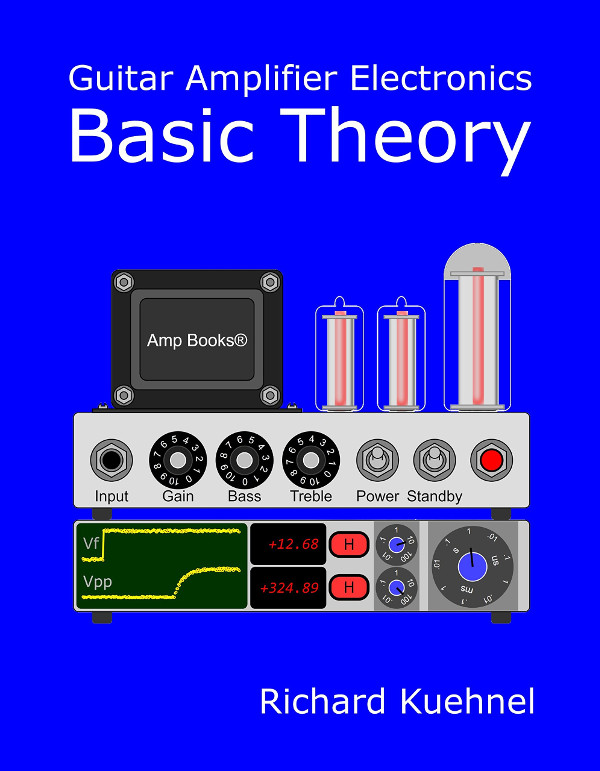Guitar Amplifier Electronics: Basic Theory - master the basics of preamp, power amp, and power supply design.

#### Multiplication

What are these products:

(2)(1) = ?
(2)(2) = ?
(2)(3) = ?

OK, we know the answers, but work with me. Let's go through the motions like we never graduated from first grade. To compute 2 times 1, align 1 on the C scale and 2 on the D scale.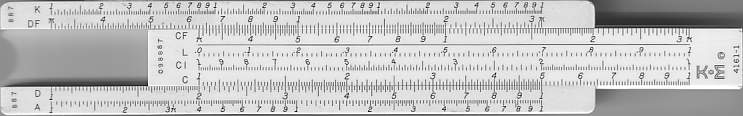Now slide the cursor over to the same point.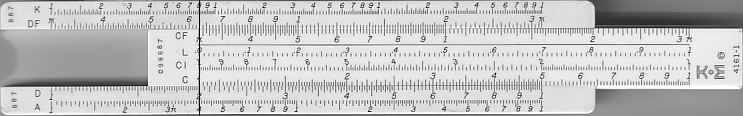Notice that the cursor now points to the answer on the D scale: 2 times 1 is 2. Now slide the cursor over to 2 on the C scale.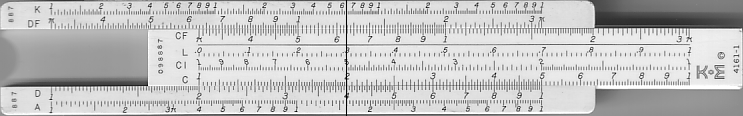It now crosses 4 on the D scale. This is the product (2)(2). Next, slide the cursor over to 3 on the C scale. It now crosses 6 on the D scale. This is equal to (2)(3). Amazing! Because the 1 on the C scale is aligned with 2 on the D scale, any number X on the C scale is aligned with 2 times X on the D scale.Fundamentals of Guitar Amplifier System Design - design your amp using a structured, professional methodology.

#### Problem

What is 3.1 times 2.6?

#### Solution

Without computing the answer exactly, we expect a number somewhere between 6 and 9. That takes care of the decimal point. Align 1 on the C scale with 31 on the D scale. Slide the cursor over to where the secondary mark on the C scale indicates the value 26. On the D scale we read the answer: somewhere between the 80 mark and the 81 mark. Based upon our preconceived notion of where the decimal point is, this puts the answer between 8.0 and 8.1.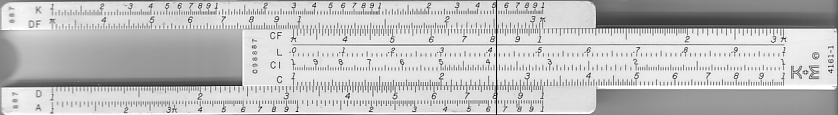The cursor looks to be about halfway between the two marks, so the answer is close to 8.05. Let's try to squeeze out a bit more accuracy. Without my bifocals this is what I see:The cursor is obviously slightly closer to 8.1 than to 8.0. It looks like 8.06 or 8.07 to me. The VLSI answer is 8.06, so on this relatively small slide rule we're getting fairly accurate results. Certainly accurate enough for analog electronics.Guitar Amplifier Electronics: Circuit Simulation - know your design works by measuring performance at every point in the amplifier.

#### Problem

What is 340 times 5.1?

#### Solution

On the low side, 300 times 5 would be 1500. On the high side, 400 times 5 would be 2000. So we're expecting a result somewhere between 1500 and 2000. That takes care of the decimal point, so now we'll let the slide rule compute the significant digits. We align 1 on the C scale with the 34 mark on the D scale. Then we swing the cursor over to 51 on the C scale and this is what we get: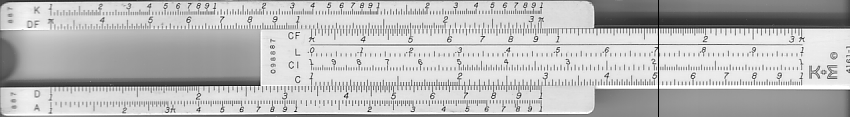We've run out of room! No need to panic. There happens to be another 1 on the C scale. The 1 at the left and the 1 at the right are called "indices" of the C scale and either can be used. We align the right index of the C scale with 34 on the D scale and slide the cursor left to 51 on the C scale to read the answer: 1730.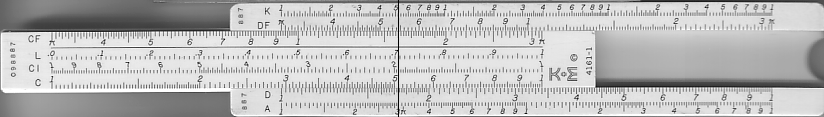The actual answer using a modern microprocessor is 1734. With a larger slide rule we could have squeezed out that extra 4 on the end, but 1730 is very close.

#### Division

Multiplication is impressive, but division rocks! Keep in mind that without transistors the alternative is long division using pencil and paper. I remember back in Mrs. Schmidt's class there was always this complicated "remainder" thing going on. Forget all that! To divide two numbers on a slide rule we simply reverse the order of the steps for multiplication. Take a look at the problem we just worked and ask yourself "what is 1734 divided by 5.1?" This is the same as saying "what number times 5.1 equals 1734?" We swing the cursor over to 1734 on the D scale (173 is close enough), which represents the known answer to the multiplication. Then we align 5.1 on the C scale with the cursor. Finally we read the answer as the number on the D scale that aligns with the index on the C scale. Putting all this together we have this:Wait a minute, that's the same image as before! Precisely. The slide and cursor are in exactly the same positions. We simply started with the known answer to a multiplication and worked our way back to the unknown value that was getting multiplied by 5.1, in this case 340.

#### Problem

What is 27100 divided by 32.5?

#### Solution

27000 divided by 30 would be 900. So we expect the answer to be slightly less than 1000. That takes care of the decimal point. We align the cursor with 271 on the D scale. Then we move the slide until 325 on the C scale aligns with the cursor. Finally we read the point on the D scale that aligns with the index of the C scale: 835. A more accurate answer, calculated by my collection of transistors from Texas Instruments, is 833.8461538. If we were living in the year 1960, and if this were the desired cathode resistor for two 12AY7 triode preamps with their cathodes connected together, then we would choose a carbon composition resistor value of 820.When more than one number is being multiplied and/or divided, the slide rule conveniently keeps track of intermediate values. Consider, for example,

(6.6)(49)/(38) = ?

We use the C and D scales to compute (6.6)(49) = 323: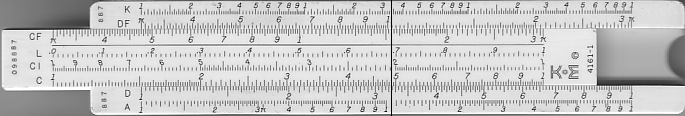Then to divide 323 by 38 we leave the cursor where it is and simply move the slide until the cursor aligns with 38 on the C scale: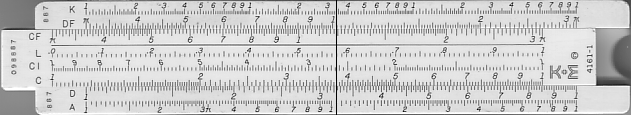Then we read 851 as the mark on the D scale that aligns with the index on the C scale. Looking at the formula we see that the answer should be somewhat higher than 6.6, so with the appropriate decimal point the result is 8.51.

#### Squares and Square Roots

The square is just a number multiplied by itself, so we could use the C and D scales to perform a multiplication. Since taking the square occurs so often, however, there's a special scale, the A scale, that performs this function for us. When the cursor is set to a number on the D scale, the square is indicated by the cursor's location on the A scale.

27.12 = ?

#### Solution

The position of the slide doesn't matter in this case. Set the cursor to 271 on the D scale and read 734 on the A scale. It doesn't get any easier than this! The actual answer, if we subject ourselves to the humility of depending on thousands of modern transistors, is 734.41. Gee, the slide rule is wrong by a whopping 0.41 - someone call the tone police!Addition and subtraction are fairly easy, so pencil and paper work fine. Multiplication is more convenient on a slide rule, but in a pinch we could do it by hand, especially if we only need a few digits accuracy. Division? Now we're getting into some serious neuron torture. A slide rule is much easier - we just do multiplication in reverse.

I don't know what elementary school is like today, but when I was in sixth grade they taught us how to compute square roots by hand - ouch! By seventh grade I had completely forgotten how it was done and in the decades since then I have never regretted my lapse of memory.

To compute a square root on a slide rule is easy - we just follow the steps of getting the square, but in reverse. Turning the previous example around, to compute the square root of 734 we place the cursor on 734 on the A scale and read the square root, 27.1 on the D scale. There's only one catch - there are two "734" positions on the A scale. This is because the actual square root depends on the location of the decimal point. The square root of 734 is 27.1. The square root of 73.4 is 8.57. The square root of 7.34 is 2.71. The square root of 0.734 is 0.857. See the pattern? If we roughly know the answer to within a factor of 10 then we know which of the two positions on the A scale to use.

#### Trigonometry and Beyond

Trigonometry, logarithms, powers of numbers - all sorts of things can be computed on the various scales of a quality slide rule. Back in the days of Leo and Jim there were special slide rules created for just about every computation imaginable. I am in possession, for example, of a vintage 1970s era slide rule for calculating system reliability based on my assumed "mean time before failure" and my desired confidence level. At the moment I'm not very "confident" I know enough about reliability to compute anything meaningful with it, but I appreciate the effort of its dedicated creators at General Dynamics Corporation.

Such were the days before digital electronics ended a slide-rule culture that put a man on the moon and gave us the 5F6-A, the Model 1987, and the DR103.New! A deep dive into the electronic design of the ultimate high-gain rig-of-death!From system design concepts to individual stage operation, an all-new examination of Bassman electronics.From "woody" to narrow panel to brownface to blackface Reverb, an in-depth exploration of Fender Deluxe circuitry.Master the basics of preamp, power amp, and power supply design.Design your amp using a structured, professional methodology.Know it works by measuring performance at every point in the amplifier.